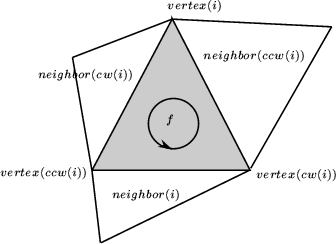## CGAL::Triangulation_cw_ccw_2

### Definition

The class Triangulation_cw_ccw_2 offer two functions int cw(int i) and int ccw(int i) which given the index of a vertex in a face compute the index of the next vertex of the same face in clockwise or counterclockwise order. This works also for neighbor indexes. Thus, for example the neighbor neighbor(cw(i)) of a face f is the neighbor which is next to neighbor(i) turning clockwise around f. The face neighbor(cw(i)) is also the first face encountered after f when turning clockwise around vertex i of f.

Many of the classes in the triangulation package inherit from Triangulation_cw_ccw_2. This is for instance the case for CGAL::Triangulation_2<Traits,Tds>::Face. Thus, for example the neighbor neighbor(cw(i)) of a face f is the neighbor which is next to neighbor(i) turning clockwise around f. The face neighbor(cw(i)) is also the first face encountered after f when turning clockwise around vertex i of f.

Figure:  Vertices and neighbors.#include <CGAL/Triangulation_2.h>

### Creation

 Triangulation_cw_ccw_2 a; default constructor.

### Operations

 int a.ccw ( const int i) returns the index of the neighbor or vertex that is next to the neighbor or vertex with index i in counterclockwise order around a face. int a.cw ( const int i) returns the index of the neighbor or vertex that is next to the neighbor or vertex with index i in counterclockwise order around a face.